## 2.2.1 Computational Effort

The computational effort of a numerical problem grows approximately linearly with the size of the problem which mainly depends on the complexity of the equation set to solve and the size of the grid. The size of the grid is directly related to the number of grid points. Due to the handling of interfaces grid points between segments contribute as well as the number of connections (lines) between the points which depend on the type of the grid elements.

Interface points are doubled in Minimos-NT (see Section 2.4). But compared to the high amount of grid points inside segments this number is normally negligible and relevant only for special devices consisting of many different segments. The lines which are the connections between grid points are processed during the matrix assembly and for pre- and post-processing.

For a tensor-product grid with an equivalent number of grid points in every direction the number of points rises with the exponent of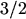when extending from two to three dimensions.

This means, that a two-dimensional grid of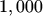points leads to a three-dimensional grid using about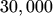points for the same resolution. Normally, two-dimensional simulations need between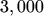andpoints. In three-dimensional space this would cover the range from about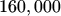to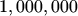points for the same resolution, which causes memory consumption which is far beyond the limits of todays, affordable computers. Therefore the necessity of an efficient grid rises dramatically in three dimensions. Nevertheless, state-of-the-art three-dimensional device simulators must be capable of handling huge amounts of simulation data.

Robert Klima 2003-02-06